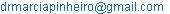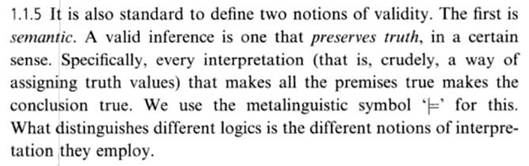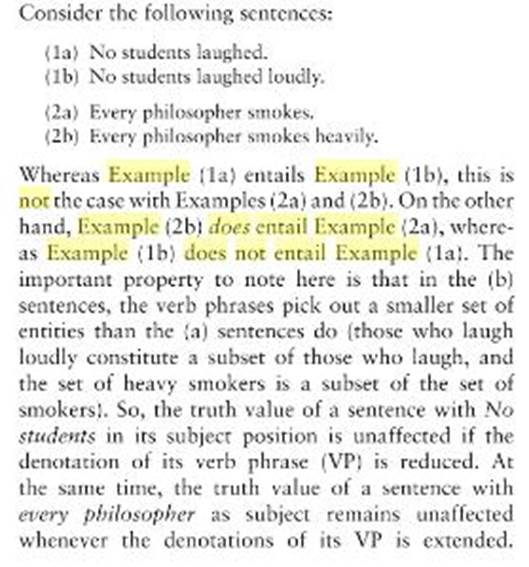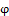Mathematics Letters
Volume 2, Issue 4, August 2016, Pages: 28-31

An Issue with the Concept of Entailment

Marcia R. Pinheiro

Department of Mathematics and Philosophy, IICSE University, Wilmington, USAMarcia R. Pinheiro. An Issue with the Concept of Entailment. Mathematics Letters. Vol. 2, No. 4, 2016, pp. 28-31. doi: 10.11648/j.ml.20160204.11

Received: September 4, 2016; Accepted: October 12, 2016; Published:October 21, 2016

Abstract: Entailment is an interesting sigmatoid: It should mean one thing, but it means another, just for starters. When used in Mathematics, it is usually with the sense of saying that something is definitely true. That would be the use in Classical Logic then. When used in Logic, it became something else. Now it was about how the logical system, which can be any nonclassical one, could be making a proposition become true or false. The major issue we found in 2000, when learning from the own nonclassicists what they do, was that they talk about Nonclassical Logic, therefore a way of thinking that is not Cartesian, yet they stick to the notion of entailment we use in Mathematics, and therefore to the Classical Logic ways. We here discuss exactly this.

Keywords: Logical System, Logic, Nonclassical, Classical, Entailment, Implication

Contents

1. Introduction

From (Pinheiro, 2016), we read the following:Figure 1. Two notions of validity, Priest.

When we have nothing to the left side followed by the symbol |=, and then a proposition, what is usually meant is that we have a tautology to the right, that is, something that is true in all interpretations that are allowed in the system (Johnstone, 1987).

Some authors, such as Johnstone (1987), say that that is the same as having {} |=, and then the proposition, what then mean that we need no propositions to support a tautology.

An interpretation is a set of truth-values being assigned to the propositions under consideration. If we have the empty set to the left side, we obviously do not have to assign truth-values to any proposition, so that the antecedent of the entailment becomes automatically true (vacuum rule perhaps).

The implication is that if we have that all the interpretations that make what is to the left side, which we are calling antecedent (Introduction, 2004), true also make what is to the right side true, and that is what we are calling consequent (Introduction, 2004), then whatever is to left entails whatever is to the right.

If the consequent is always true for any interpretation we choose, what has to be true for tautologies, and there is no interpretation to be considered in terms of antecedent, we should probably have a conflict, but the decision is that in vacuum, OK. That probably comes from the fact that for us to falsify an implication in Classical Logic (CL), there is only one way: antecedent is true, consequent is false. If we guarantee that the consequent is true, then the implication will always be true, like regardless of the antecedent.

Nonclassical Logic has been created by the revolutionary: They wanted CL to be used ALSO in real life. In real life, people have doubts, so that something might be true, and false at the same time for at least some amount of time. True, and false were not enough in this case.

Nonclassical Logic came to pervert the rules from Classical Logic, therefore, to basically promote revolution in it: Why are you Cartesian in what regards gender? We have men, women, and middle-sex. When we want to assign a truth-value to a sentence of the type I am a woman, we will be in trouble if the person is from the middle-sex. Accept a maybe instead of a true or false, and it is all fixed.

Another scholar found a fourth way to go, and there was another system, Fuzzy Logic (Rouse, 2016) came up with infinitely many, and so on.

What we find most interesting however is that they want to use, according to Priest (2001), the same notion of entailment that we use in Mathematics, and therefore in CL. Do half the work, and say you did the same, basically.

We think we are sure they should at least have thought for longer about it, so perhaps they should have produced a paper on the topic, some book chapters, and whatever else to show that they have thought about it.

Intuitively, the concept of entailment would have to adapt to the logical system we have at hand, so that if we have a three-valued system, so say that we have the truth-values A, B, and C, and rules that perhaps agglutinate to the left side of the entailment, so say two values to the left give the same result in the end if the right side keeps its value, so say A, and B to one side, and A to the other would still give us OK or A, we would have to at least write that in the definition of entailment, it seems. In this case, instead of whatever interpretation makes the left side become true, we should have whatever interpretation makes the left side become true or middle-value, like to the least.

In this paper, we try to discuss this interesting concept, of entailment, and propose that nonclassicists think more about the topic or expose what they have already discussed in a way for us to understand that they have already thought enough about it.

2. Development

So, if we had only one proposition, say P: x belongs to the reals, and we wanted to know if that entailed that Q: x+2=5 => x=3, we would have to play the following game: Assume that v(P) = 1. Now we know that x does belong to the reals. With this, we know that v(Q) = 1 as well. Therefore, P |= Q.

If instead we had P: x belongs to the interval (7,10), we would know that Q would be true in the same way because both antecedent, and consequent of the implication would be false, what gives us a true implication, so that we would have v(P)=1 => v(Q)=1, and therefore P |= Q.

That is counter-intuitive in all. Perhaps we should revise the definition of entailment even in terms of Classical Logic.

Some people have discussed semantic intersection/connection in terms of entailment, and even implication (Mares, 1998) at waste, however, and nothing that could add seems to have been found.

It makes some sense to believe that if by making the left side true we get the right side true, then we have an entailment.

Entailment is defined in the following way (Harper, 2001):Figure 2. Etymology of the word Entailment.

We then understand that it should mean the creation of a legal limitation. Since the logical system has rules, we can take those to be laws, and then say that if A entails B, then A provokes the appearance of a limitation for B.

If nothing appears to the left side, we understand there are no limitations, and that is when we say we have a tautology to the right side of the symbol |=, so that it is all making a lot of sense so far.

If this is the actual sense of entails, then the nonclassicists could be right in using it for their nonclassical systems without adapting or changing anything, but they then would probably have to stop saying that whatever makes the left side true would have to make the right side true.

The right way of putting it would probably be: If the left side does not bring any opposition to whatever is on the right side, then we have an entailment.

Now we can accept that any contradiction, and nothing would be the same, since it would not impose any limitation to the right side. Up to this date, however, we thought that there was a bit of confusion, since contradictions would not be true in CL.

If the left side said that A was zero, so say A=0, then the right side could not bring A=2, we assume.

There is a bit of confusion in the literature, however.

Allan (2010) let us know thatFigure 3. Encyclopedia, Entails, definition.

In this case, the source claims that (1b) does not entail (1a). (1b) says that no students laughed loudly, and (1a) says that no students laughed. If no students laughed loudly, we are not saying that no student laughed, quite trivially, but (1a) would be a restriction on (1b), a limitation, like we would have reduced the group of students that laughed even further, so that, in our point of view, that would have been a limitation. (1b) does not limit (1a), it is the opposite, so that we here would think that all is agreeing with the just proposed new definition for entailment, since (1a) does entail (1b) according to the source.

It also claims that (2a) does not entail (2b). (2b) says that every philosopher smokes heavily. (2a) says that every philosopher smokes. (2b) would clearly be a restriction on (2a), therefore a limitation, so that (2b) does entail (2a). On the other hand, (2a) would not entail (2b) if we consider our new definition, since (2a) is not limiting (2b), it is the opposite.

The source seems to have the same understanding we just acquired here: Limitations are what entails, a reduction in the domain provoked by the left side of the relationship we analyse.

From (Stanford, 2016), comes the following extract:

Logical Entailment

We say that a sentencelogically entails a sentence ψ (written|= ψ) if and only if every truth assignment that satisfiesalso satisfies ψ. More generally, we say that a set of sentences Δ logically entails a sentence ψ (written Δ |= ψ) if and only if every truth assignment that satisfies all of the sentences in Δ also satisfies ψ.

For example, the sentence p logically entails the sentence (p q). Since a disjunction is true whenever one of its disjuncts is true, then (p q) must be true whenever p is true. On the other hand, the sentence p does not logically entail (p q). A conjunction is true if and only if both of its conjuncts are true, and q may be false. Of course, any set of sentences containing both p and q does logically entail (p q).

Note that the relationship of logical entailment is a purely logical one. Even if the premises of a problem do not logically entail the conclusion, this does not mean that the conclusion is necessarily false, even if the premises are true. It just means that it is possible that the conclusion is false.

Once again, consider the case of (p q). Although p does not logically entail this sentence, it is possible that both p and q are true and, therefore, (p q) is true. However, the logical entailment does not hold because it is also possible that q is false and, therefore, (p q) is false.

Note also that logical entailment is not the same as logical equivalence. The sentence p logically entails (p q), but (p q) does not logically entail p. Logical entailment is not analogous to arithmetic equality; it is closer to arithmetic inequality.

This definition matches that of Dr. Priest (2001), and fellows, but is completely different from the definition we see in (Allan, 2010).

So, they say that p entails p or q. We may think that we can replace their definition of entailment with an implication: p -> p or q. Notwithstanding, seeing things from closer, we would get antecedent false validating the implication as well, but they reduce it all to antecedent true with consequent true, so that we only have one case of the three allowed cases in the implication when it comes to their entailment: It is something apart.

Harper (2001) gives us the sense have consequences or inseparable connection as an alternative, and he mentions that this sense appeared in the year of 1829.

If the sense is to have consequences, then the left side of the entailment would have as a consequence the right side, what is then compatible with the definition we find in Stanford (2016), and is also compatible with the definition we find in (Allan, 2010), considering the examples he there gives.

Now, this thing of being true to one side leading to all being true to the other or not imposing a situation in which the other side would not be true, is actually connected to the only way to falsify an implication in CL, which is antecedent true when consequent isn’t.

We are only using two possible truth-values here, true, and false, and therefore we are obviously using CL.

Notwithstanding, Fuzzy Logic would have an infinity of possible truth-values, and we perhaps would need to consider those when talking about entailment inside of that system. That is actually the point of this paper.

As we go from CL to nonclassical systems, we should probably also find new definitions for entailment, and not only for validity.

Hajek (2002) says that

The standard set of truth degrees is the real interval [0, 1] with its natural ordering ≤ (1 standing for absolute truth, 0 for absolute falsity); but one can work with different domains, finite or infinite, linearly or partially ordered. Truth functions of connectives have to behave classically on the extremal values 0,1.

He is talking about Fuzzy Logic.

We read (Priest, 2001), and found only one definition of entailment, which is the one we present here. It is possible that that is wrong because if you are changing your truth values, and instead of two you now have even infinitely many, you would have to change the way you think of entailment for it all to make sense, like true, and false was for CL.

We could then have, in the case of Fuzzy Logic, that whatever makes the left side receive a truth-value between 0.5, and 1 makes the right side receive a truth-value between 0.5, and 1 instead.

We feel that what they shouldn’t have changed they did change, which is the concept of Completeness (Pinheiro, 2016), and what they should have changed, they didn’t change, which is the concept of entailment.

3. Conclusion

Nonclassicists would have to come up with articles to at least justify their choices in terms of the definition of entailment: If they change truth-values, that should provoke a change also in terms of the definition of entailment, since that definition comes attached to truth-values. The definition says that whatever makes the antecedent true would have to make the consequent true for us to have an entailment.

It is possible that they would have to present a new definition of entailment for each nonclassical system that be not bivalent.

Here we have the opposite to what we had when we talked about Completeness (Pinheiro, 2016): We should be changing everything that has been created for CL.

Not entering details on how Fuzzy Logic has been used or defined so far, just talking about truth-values, it could be that we would have to say that entailment in Fuzzy Logic is only justified if both antecedent, and consequent are marked with a value that is between 0.5, and 1.

It seems that the best translation for the symbol |= is have consequences or inseparable connection. Perhaps the best way to word it would be has as a consequence.

In this way, if X |= Y, X has, as a consequence, Y.

This is something different from the implication because, in terms of CL, for instance, if the antecedent of the implication is evaluated as false, and the consequent as true, the implication is true, but, with the entailment, we only accept true to both sides as a way to validate the relationship.

References

1. Pinheiro, M. R. (2016). Completeness. IOSR - Jornal of Mathematics, 12(5), 34–37. Retrieved from
https://www.researchgate.net/publication/307122839_Completeness
2. Johnstone, P. T. (1987). Notes on Logic and Set Theory. Cambridge University Press. Retrieved from
A&hl=en&sa=X&ved=0ahUKEwj1vsrXsfDOAhXG7hoKHSguAsw4KBDoAQgaMAA#v=onepage&q=logical entailment tautology&f=false
3. Rouse, M. (2016). Fuzzy Logic. Retrieved September 27, 2016, from http://whatis.techtarget.com/definition/fuzzy-logic
4. Priest, G. (2001). An Introduction to Non-Classical Logic. Cambridge University Press.
5. Mares, E. (1998). Relevance Logic. Retrieved October 15, 2016, from http://plato.stanford.edu/entries/logic-relevance/
6. Harper, D. (2001). Entail. Retrieved September 3, 2016, from http://www.etymonline.com/index.php?search=Entailment
7. Allan, K. (2010). Concise Encyclopedia of Semantics. Elsevier. Retrieved from
https://books.google.com.au/books?id=3_1snsgmqU8C&pg=PA561&lpg=PA561&dq=does+not+entail+example+logic&source=bl&ots=jAX-weH0ml&sig=CPfESWbKBv7_sO8ABAz1H5xREDM&hl=en&sa=X&ved=0ahUKEwiX1LSOn_POAhXCE5QKHXf2Afo4ChDoAQgeMAE#v=onepage&q=does not entail example logic&f=false
8. Stanford Logic Group. (2016). Logical Properties and Relationships. Retrieved September 3, 2016, from
http://logic.stanford.edu/intrologic/notes/chapter_03.html
9. Hajek, P. (2002). Fuzzy Logic. Retrieved September 4, 2016, fromhttp://plato.stanford.edu/entries/logic-fuzzy/
10. Introduction to Logic. (2004). Retrieved September 4, 2016, from http://philosophy.lander.edu/logic/conditional.html

 Contents 1. 2. 3.
Article ToolsAbstractPDF(368K)